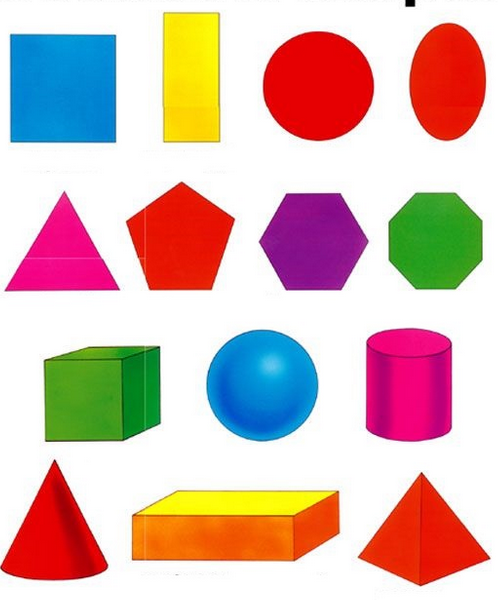# Describing Shapes

Directions: Using the following picture, complete the following sentences (using the phrases: above, below, beside, in front of, behind, and next to)The cube is ___________ the sphere and ___________ the triangle.

The hexagon is __________ the pentagon and __________ the circle.

Use the shape names to complete the following statements:

The ________ is next to the ________ and above the __________.

The ________ is beside the __________, above the ___________, and below the ___________.

### Hint

Answers can vary, one possible solution is:
The cube is next to the sphere and below the triangle.

The hexagon is beside the pentagon and below the circle.

The rectangle is next to the square and above the pentagon.

The octagon is beside the hexagon, above the cylinder, and below the oval (ellipse).

Source: Bryan Anderson

## Adding and Subtracting Within 10

Directions: Using the digits 1 to 9 at most one time each, place a digit …

1.Christina Chatzis

1. Next to and below,
2. Beside and below
3. Circle and sphere pyramid
4 triangle, hexagon, cube, square

2.Calvin Petruccelli

1 next to, below

2 next to, below

3 square, rectangle, triangle

4 pentagon, hexagon, , cube, rectangle

3.beside, below
beside, below
triangle, pentagon, cube
sphere, cylinder, cuboid

4.1. Next to, below
2. Beside, below
3. Circle, rectangle, hexagon
4. Cube, sphere, Cone, triangle

5.next to, below
beside, below
sphere, cylinder, rectangular prism
sphere, cube, rectangular prism, hexagon

6.The cube is ____next to______ the sphere and ___below________ the triangle.

The hexagon is ___next to_______ the pentagon and __below________ the circle.

Use the shape names to complete the following statements:

The ___sphere_____ is next to the __cube______ and above the ____rectangle______.

The ___cube_____ is beside the ___sphere_______, above the ___cone________, and below the ___triangle________.

7.1) next to, below
2) beside, below
3) cube, sphere, cone
4)cylinder, sphere, pyramid

8.1. Next to, below
2. beside, below
3. cube sphere, cone
4. cylinder, sphere, pyramid

9.next to / below
next to / below
triangle / pentagon / cube
octagon / hexagon / cylinder / oval

10.1.next to, below
2. Next to, below
3.cube sphere cone
4. Octagon, hexagon, cylinder,oval

11.1 next to below
2.beside below
3c.ube sphere cone’4.cylinder sphere, pyramid

12.1. Next to, below
2. Next to, below
3. Square, Rectangle, Triangle
4. Triangle, Pentagon, Cube, Square

13.Nicholas Mendoza

1) next to, below
2) next to, below
3) circle, oval, hexagon
4) cube, sphere, cone, triangle

14.The cube is next to the square and below the triangle.

The hexagon is next to the pentagon and below the circle.

The sphere is next to the cube and above the rectangle.

The hexagon is beside the octagon above the cylinder and below the oval.

15.This is not only good for Kinder math but also covers Social Studies standards for Kinder.